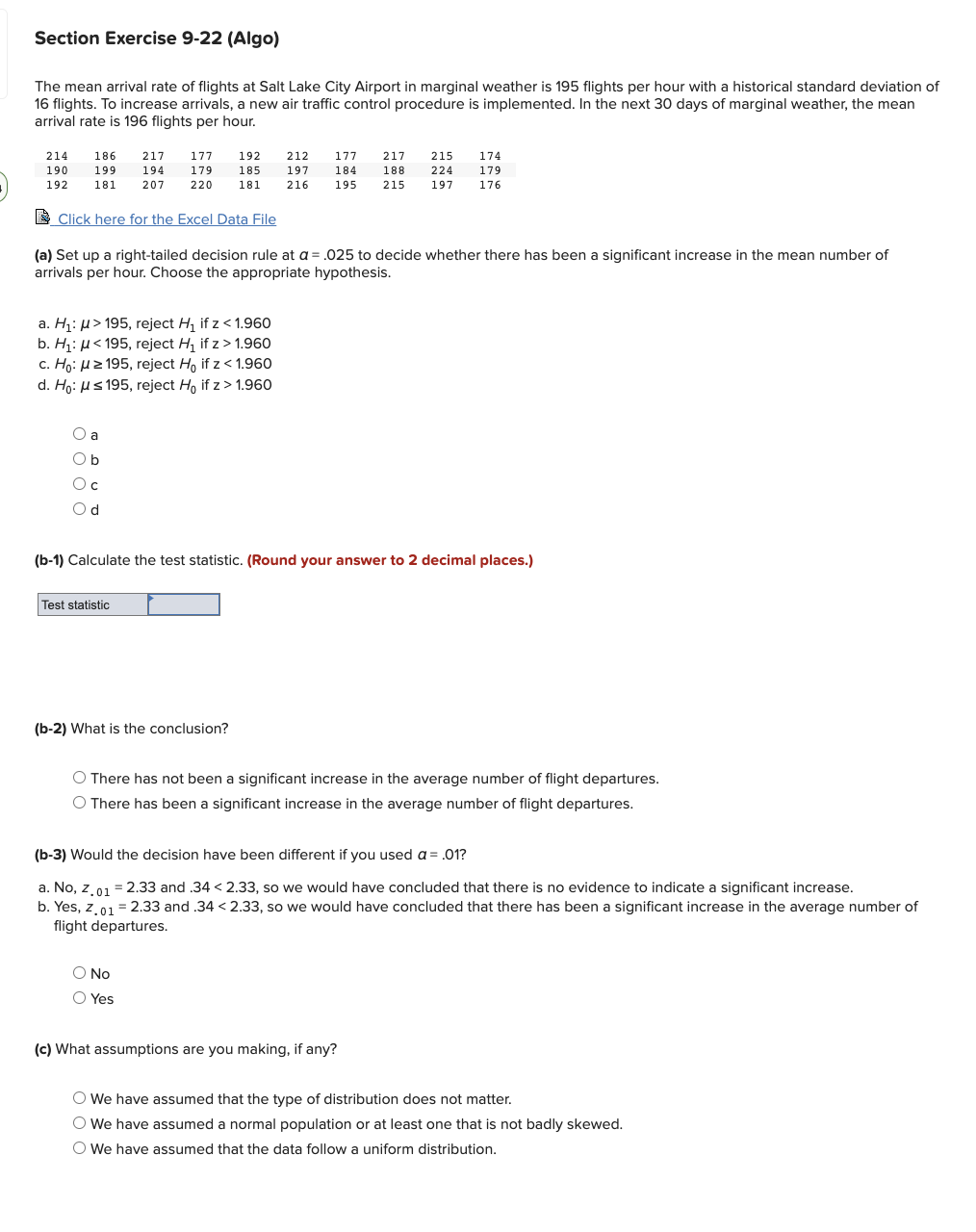Home / Expert Answers / Statistics and Probability / the-mean-arrival-rate-of-flights-at-salt-lake-city-airport-in-marginal-weather-is-195-flights-per-pa631

# (Solved): The mean arrival rate of flights at Salt Lake City Airport in marginal weather is 195 flights per ...The mean arrival rate of flights at Salt Lake City Airport in marginal weather is 195 flights per hour with a historical standard deviation of 16 flights. To increase arrivals, a new air traffic control procedure is implemented. In the next 30 days of marginal weather, the mean arrival rate is 196 flights per hour. ? Click here for the Excel Data File (a) Set up a right-tailed decision rule at $$a=.025$$ to decide whether there has been a significant increase in the mean number of arrivals per hour. Choose the appropriate hypothesis. a. $$H_{1}: \mu>195$$, reject $$H_{1}$$ if $$z<1.960$$ b. $$H_{1}: \mu<195$$, reject $$H_{1}$$ if $$z>1.960$$ c. $$H_{0}: \mu \geq 195$$, reject $$H_{0}$$ if $$z<1.960$$ d. $$H_{0}: \mu \leq 195$$, reject $$H_{0}$$ if $$z>1.960$$ (b-1) Calculate the test statistic. (Round your answer to 2 decimal places.) (b-2) What is the conclusion? There has not been a significant increase in the average number of flight departures. There has been a significant increase in the average number of flight departures. (b-3) Would the decision have been different if you used $$a=.01 ?$$ a. No, $$z_{.01}=2.33$$ and $$.34<2.33$$, so we would have concluded that there is no evidence to indicate a significant increase. b. Yes, $$z .01=2.33$$ and $$.34<2.33$$, so we would have concluded that there has been a significant increase in the average number of flight departures. No Yes (c) What assumptions are you making, if any? We have assumed that the type of distribution does not matter. We have assumed a normal population or at least one that is not badly skewed. We have assumed that the data follow a uniform distribution.

We have an Answer from Expert